# sfepy.terms.terms_jax module¶

Proof-of-concept JAX-based terms supporting automatic differentiation.

class sfepy.terms.terms_jax.LinearElasticLADTerm(name, arg_str, integral, region, **kwargs)[source]

Homogeneous isotropic linear elasticity term differentiable w.r.t. material parameters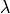,(Lamé’s parameters).

Definition: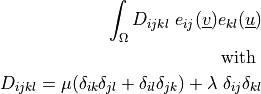Call signature:

 dw_lin_elastic_l_ad (material_1, material_2, virtual, state)
Arguments:
• material_1:(Lamé’s first parameter)

• material_2:(Lamé’s second parameter, shear modulus)

• virtual/parameter_1: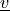• state/parameter_2: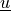arg_shapes = {'material_1': '1, 1', 'material_2': '1, 1', 'state': 'D', 'virtual': ('D', 'state')}
arg_types = (('material_1', 'material_2', 'virtual', 'state'),)
diff_info = {'material_1': 1, 'material_2': 1}
static function(out, fun, fargs)[source]
get_fargs(material1, material2, virtual, state, mode=None, term_mode=None, diff_var=None, **kwargs)[source]
modes = ('weak',)
class sfepy.terms.terms_jax.LinearElasticYPADTerm(name, arg_str, integral, region, **kwargs)[source]

Homogeneous isotropic linear elasticity term differentiable w.r.t. material parameters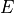(Young’s modulus),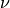(Poisson’s ratio).

Definition:Call signature:

 dw_lin_elastic_yp_ad (material_1, material_2, virtual, state)
Arguments:
• material_1:• material_2:• virtual/parameter_1:• state/parameter_2:arg_shapes = {'material_1': '1, 1', 'material_2': '1, 1', 'state': 'D', 'virtual': ('D', 'state')}
arg_types = (('material_1', 'material_2', 'virtual', 'state'),)
diff_info = {'material_1': 1, 'material_2': 1}
static function(out, fun, fargs)[source]
get_fargs(material1, material2, virtual, state, mode=None, term_mode=None, diff_var=None, **kwargs)[source]
modes = ('weak',)
class sfepy.terms.terms_jax.MassADTerm(name, arg_str, integral, region, **kwargs)[source]

Homogeneous mass term differentiable w.r.t. the material parameter.

Definition: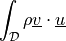Call signature:

 dw_mass_ad (material, virtual, state)
Arguments:
• material_1: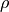• virtual:• state:arg_shapes = {'material': '1, 1', 'state': 'D', 'virtual': ('D', 'state')}
arg_types = (('material', 'virtual', 'state'),)
diff_info = {'material': 1}
static function(out, fun, fargs)[source]
get_fargs(material_density, virtual, state, mode=None, term_mode=None, diff_var=None, **kwargs)[source]
modes = ('weak',)
sfepy.terms.terms_jax.ceval_elasticity_l(lam, mu, vbfg, ubfg, det, cu)[source]
sfepy.terms.terms_jax.ceval_elasticity_yp(young, poisson, plane, vbfg, ubfg, det, cu)[source]
sfepy.terms.terms_jax.ceval_mass(density, vbf, ubf, det, cu)[source]
sfepy.terms.terms_jax.eval_density_mass(density, vbf, ubf, det, cu)

Vectorized version of ceval_mass. Takes similar arguments as ceval_mass but with additional array axes over which ceval_mass is mapped.

Original documentation:

Jacobian of ceval_mass with respect to positional argument(s) 0. Takes the same arguments as ceval_mass but returns the jacobian of the output with respect to the arguments at positions 0.

sfepy.terms.terms_jax.eval_elasticity_l(lam, mu, vbfg, ubfg, det, cu)

Vectorized version of ceval_elasticity_l. Takes similar arguments as ceval_elasticity_l but with additional array axes over which ceval_elasticity_l is mapped.

sfepy.terms.terms_jax.eval_elasticity_yp(young, poisson, plane, vbfg, ubfg, det, cu)

Vectorized version of ceval_elasticity_yp. Takes similar arguments as ceval_elasticity_yp but with additional array axes over which ceval_elasticity_yp is mapped.

sfepy.terms.terms_jax.eval_jac_elasticity_l(lam, mu, vbfg, ubfg, det, cu)

Vectorized version of ceval_elasticity_l. Takes similar arguments as ceval_elasticity_l but with additional array axes over which ceval_elasticity_l is mapped.

Original documentation:

Jacobian of ceval_elasticity_l with respect to positional argument(s) -1. Takes the same arguments as ceval_elasticity_l but returns the jacobian of the output with respect to the arguments at positions -1.

sfepy.terms.terms_jax.eval_jac_elasticity_yp(young, poisson, plane, vbfg, ubfg, det, cu)

Vectorized version of ceval_elasticity_yp. Takes similar arguments as ceval_elasticity_yp but with additional array axes over which ceval_elasticity_yp is mapped.

Original documentation:

Jacobian of ceval_elasticity_yp with respect to positional argument(s) -1. Takes the same arguments as ceval_elasticity_yp but returns the jacobian of the output with respect to the arguments at positions -1.

sfepy.terms.terms_jax.eval_jac_mass(density, vbf, ubf, det, cu)

Vectorized version of ceval_mass. Takes similar arguments as ceval_mass but with additional array axes over which ceval_mass is mapped.

Original documentation:

Jacobian of ceval_mass with respect to positional argument(s) -1. Takes the same arguments as ceval_mass but returns the jacobian of the output with respect to the arguments at positions -1.

sfepy.terms.terms_jax.eval_lam_elasticity_l(lam, mu, vbfg, ubfg, det, cu)

Vectorized version of ceval_elasticity_l. Takes similar arguments as ceval_elasticity_l but with additional array axes over which ceval_elasticity_l is mapped.

Original documentation:

Jacobian of ceval_elasticity_l with respect to positional argument(s) 0. Takes the same arguments as ceval_elasticity_l but returns the jacobian of the output with respect to the arguments at positions 0.

sfepy.terms.terms_jax.eval_mass(density, vbf, ubf, det, cu)

Vectorized version of ceval_mass. Takes similar arguments as ceval_mass but with additional array axes over which ceval_mass is mapped.

sfepy.terms.terms_jax.eval_mu_elasticity_l(lam, mu, vbfg, ubfg, det, cu)

Vectorized version of ceval_elasticity_l. Takes similar arguments as ceval_elasticity_l but with additional array axes over which ceval_elasticity_l is mapped.

Original documentation:

Jacobian of ceval_elasticity_l with respect to positional argument(s) 1. Takes the same arguments as ceval_elasticity_l but returns the jacobian of the output with respect to the arguments at positions 1.

sfepy.terms.terms_jax.eval_poisson_elasticity_yp(young, poisson, plane, vbfg, ubfg, det, cu)

Vectorized version of ceval_elasticity_yp. Takes similar arguments as ceval_elasticity_yp but with additional array axes over which ceval_elasticity_yp is mapped.

Original documentation:

Jacobian of ceval_elasticity_yp with respect to positional argument(s) 1. Takes the same arguments as ceval_elasticity_yp but returns the jacobian of the output with respect to the arguments at positions 1.

sfepy.terms.terms_jax.eval_young_elasticity_yp(young, poisson, plane, vbfg, ubfg, det, cu)

Vectorized version of ceval_elasticity_yp. Takes similar arguments as ceval_elasticity_yp but with additional array axes over which ceval_elasticity_yp is mapped.

Original documentation:

Jacobian of ceval_elasticity_yp with respect to positional argument(s) 0. Takes the same arguments as ceval_elasticity_yp but returns the jacobian of the output with respect to the arguments at positions 0.

sfepy.terms.terms_jax.get_strain(gu)[source]
sfepy.terms.terms_jax.get_stress(lam, mu, gu)[source]